# Lecture-4 Reduction of Quadratic Form.pdf

29 May 2023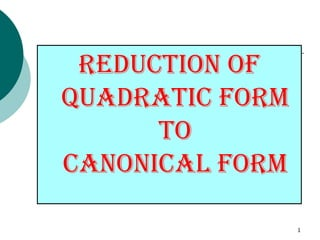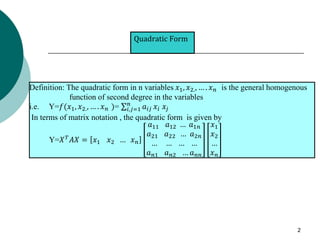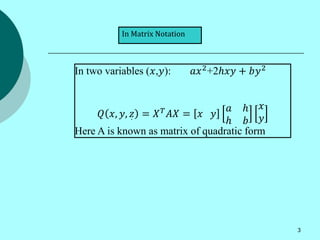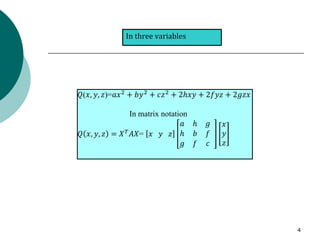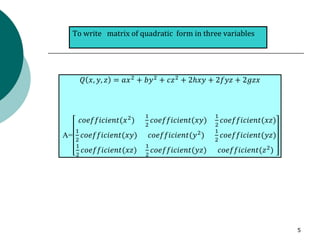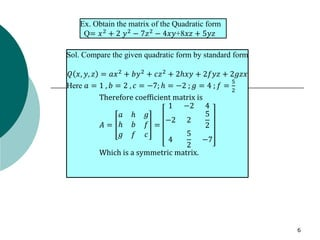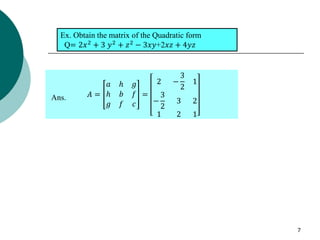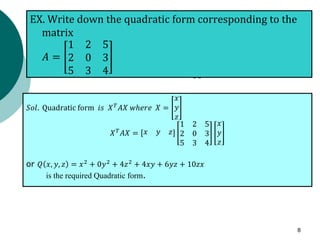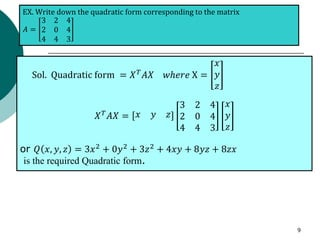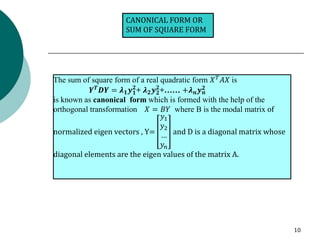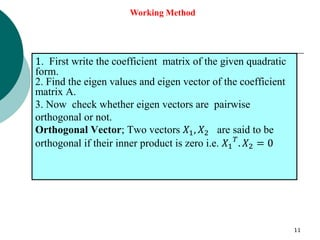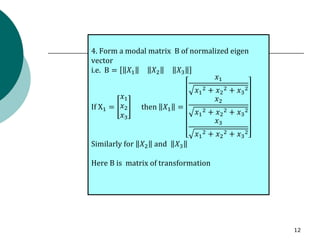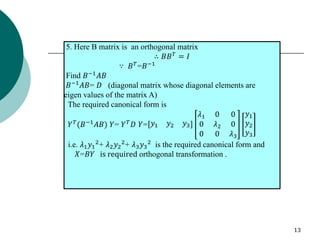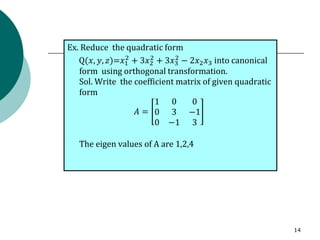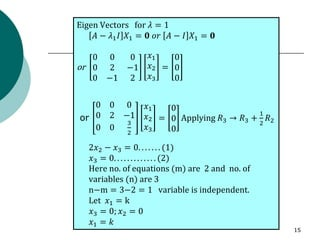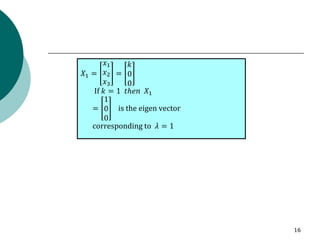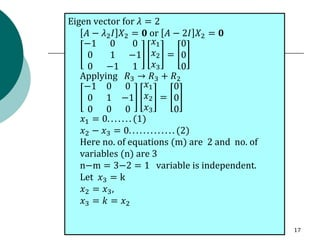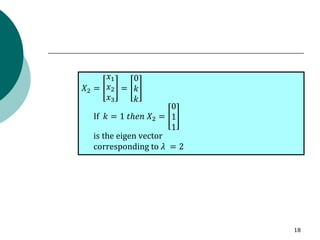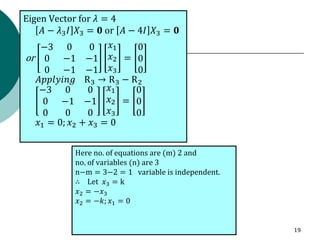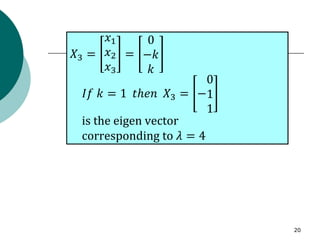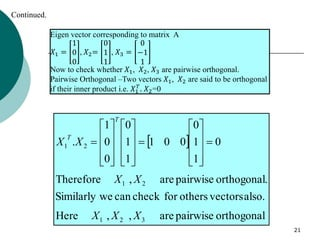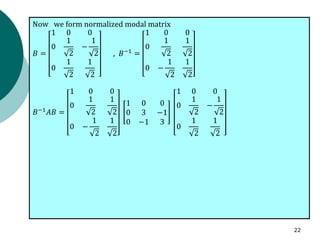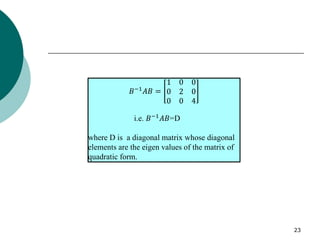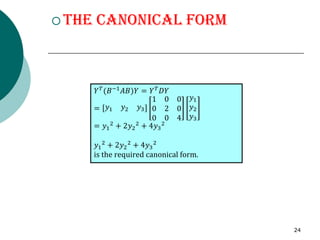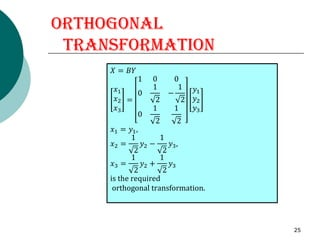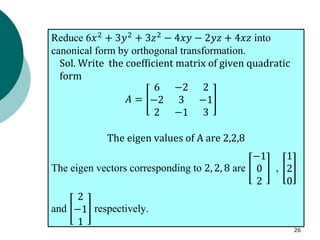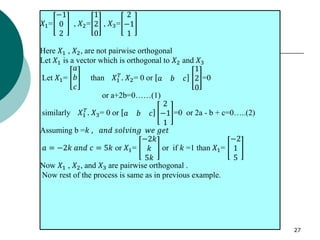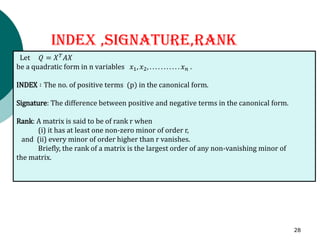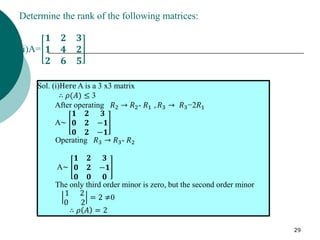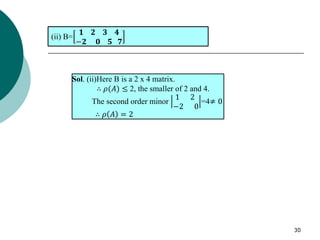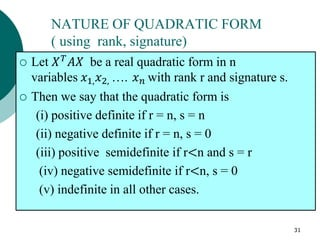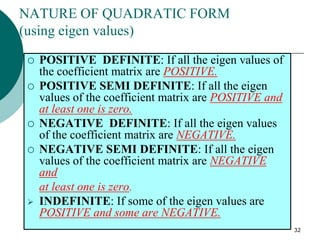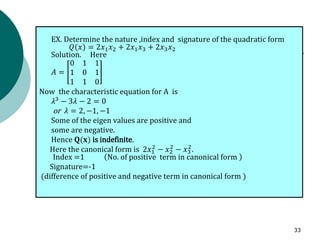1 sur 33

### Lecture-4 Reduction of Quadratic Form.pdf

• 2. Quadratic Form 2 Definition: The quadratic form in n variables 𝑥1, 𝑥2., … . 𝑥𝑛 is the general homogenous function of second degree in the variables i.e. Y=𝑓(𝑥1, 𝑥2., … . 𝑥𝑛 )= σ𝑖,𝑗=1 𝑛 𝑎𝑖𝑗 𝑥𝑖 𝑥𝑗 In terms of matrix notation , the quadratic form is given by Y=𝑋𝑇 𝐴𝑋 = 𝑥1 𝑥2 … 𝑥𝑛 𝑎11 𝑎12 … 𝑎1𝑛 𝑎21 𝑎22 … 𝑎2𝑛 … … … … 𝑎𝑛1 𝑎𝑛2 … 𝑎𝑛𝑛 𝑥1 𝑥2 … 𝑥𝑛
• 3. In Matrix Notation 3 In two variables (𝑥,𝑦): 𝑎𝑥2+2ℎ𝑥𝑦 + 𝑏𝑦2 𝑄 𝑥, 𝑦, 𝑧 = 𝑋𝑇𝐴𝑋 = 𝑥 𝑦 𝑎 ℎ ℎ 𝑏 𝑥 𝑦 Here A is known as matrix of quadratic form .
• 4. In three variables 4 𝑄(𝑥, 𝑦, 𝑧)=𝑎𝑥2 + 𝑏𝑦2 + 𝑐𝑧2 + 2ℎ𝑥𝑦 + 2𝑓𝑦𝑧 + 2𝑔𝑧𝑥 In matrix notation 𝑄 𝑥, 𝑦, 𝑧 = 𝑋𝑇 𝐴𝑋= 𝑥 𝑦 𝑧 𝑎 ℎ 𝑔 ℎ 𝑏 𝑓 𝑔 𝑓 𝑐 𝑥 𝑦 𝑧
• 5. To write matrix of quadratic form in three variables 5 𝑄 𝑥, 𝑦, 𝑧 = 𝑎𝑥2 + 𝑏𝑦2 + 𝑐𝑧2 + 2ℎ𝑥𝑦 + 2𝑓𝑦𝑧 + 2𝑔𝑧𝑥 A= 𝑐𝑜𝑒𝑓𝑓𝑖𝑐𝑖𝑒𝑛𝑡(𝑥2 ) 1 2 𝑐𝑜𝑒𝑓𝑓𝑖𝑐𝑖𝑒𝑛𝑡(𝑥𝑦) 1 2 𝑐𝑜𝑒𝑓𝑓𝑖𝑐𝑖𝑒𝑛𝑡(𝑥𝑧) 1 2 𝑐𝑜𝑒𝑓𝑓𝑖𝑐𝑖𝑒𝑛𝑡(𝑥𝑦) 𝑐𝑜𝑒𝑓𝑓𝑖𝑐𝑖𝑒𝑛𝑡(𝑦2) 1 2 𝑐𝑜𝑒𝑓𝑓𝑖𝑐𝑖𝑒𝑛𝑡(𝑦𝑧) 1 2 𝑐𝑜𝑒𝑓𝑓𝑖𝑐𝑖𝑒𝑛𝑡(𝑥𝑧) 1 2 𝑐𝑜𝑒𝑓𝑓𝑖𝑐𝑖𝑒𝑛𝑡(𝑦𝑧) 𝑐𝑜𝑒𝑓𝑓𝑖𝑐𝑖𝑒𝑛𝑡(𝑧2 )
• 6. 6 Ex. Obtain the matrix of the Quadratic form Q= 𝑥2 + 2 𝑦2 − 7𝑧2 − 4𝑥𝑦+8𝑥𝑧 + 5𝑦𝑧 Sol. Compare the given quadratic form by standard form 𝑄 𝑥, 𝑦, 𝑧 = 𝑎𝑥2 + 𝑏𝑦2 + 𝑐𝑧2 + 2ℎ𝑥𝑦 + 2𝑓𝑦𝑧 + 2𝑔𝑧𝑥 Here 𝑎 = 1 , 𝑏 = 2 , 𝑐 = −7; ℎ = −2 ; 𝑔 = 4 ; 𝑓 = 5 2 Therefore coefficient matrix is 𝐴 = 𝑎 ℎ 𝑔 ℎ 𝑏 𝑓 𝑔 𝑓 𝑐 = 1 −2 4 −2 2 5 2 4 5 2 −7 Which is a symmetric matrix.
• 7. 7 Ex. Obtain the matrix of the Quadratic form Q= 2𝑥2 + 3 𝑦2 + 𝑧2 − 3𝑥𝑦+2𝑥𝑧 + 4𝑦𝑧 𝐴 = 𝑎 ℎ 𝑔 ℎ 𝑏 𝑓 𝑔 𝑓 𝑐 = 2 − 3 2 1 − 3 2 3 2 1 2 1 Ans.
• 8. 𝐴 = EX. Write down the quadratic form corresponding to the matrix 𝐴 = 1 2 5 2 0 3 5 3 4 𝑆𝑜𝑙. Quadratic form 𝑖𝑠 𝑋𝑇 𝐴𝑋 𝑤ℎ𝑒𝑟𝑒 𝑋 = 𝑥 𝑦 𝑧 𝑋𝑇 𝐴𝑋 = 𝑥 𝑦 𝑧 1 2 5 2 0 3 5 3 4 𝑥 𝑦 𝑧 or 𝑄 𝑥, 𝑦, 𝑧 = 𝑥2 + 0𝑦2 + 4𝑧2 + 4𝑥𝑦 + 6𝑦𝑧 + 10𝑧𝑥 is the required Quadratic form. 8
• 9. Sol. Quadratic form = 𝑋𝑇 𝐴𝑋 𝑤ℎ𝑒𝑟𝑒 X = 𝑥 𝑦 𝑧 𝑋𝑇 𝐴𝑋 = 𝑥 𝑦 𝑧 3 2 4 2 0 4 4 4 3 𝑥 𝑦 𝑧 or 𝑄 𝑥, 𝑦, 𝑧 = 3𝑥2 + 0𝑦2 + 3𝑧2 + 4𝑥𝑦 + 8𝑦𝑧 + 8𝑧𝑥 is the required Quadratic form. EX. Write down the quadratic form corresponding to the matrix 𝐴 = 3 2 4 2 0 4 4 4 3 9
• 10. CANONICAL FORM OR SUM OF SQUARE FORM 10 The sum of square form of a real quadratic form 𝑋𝑇 𝐴𝑋 is 𝒀𝑻𝑫𝒀 = 𝝀𝟏𝒚𝟏 𝟐 + 𝝀𝟐𝒚𝟐 𝟐 +…… +𝝀𝒏𝒚𝒏 𝟐 is known as canonical form which is formed with the help of the orthogonal transformation 𝑋 = 𝐵𝑌 where B is the modal matrix of normalized eigen vectors , Y= 𝑦1 𝑦2 … 𝑦𝑛 and D is a diagonal matrix whose diagonal elements are the eigen values of the matrix A.
• 11. 11 1. First write the coefficient matrix of the given quadratic form. 2. Find the eigen values and eigen vector of the coefficient matrix A. 3. Now check whether eigen vectors are pairwise orthogonal or not. Orthogonal Vector; Two vectors 𝑋1, 𝑋2 are said to be orthogonal if their inner product is zero i.e. 𝑋1 𝑇 . 𝑋2 = 0 Working Method
• 12. 12 4. Form a modal matrix B of normalized eigen vector i.e. B = 𝑋1 𝑋2 𝑋3 If X1 = 𝑥1 𝑥2 𝑥3 then 𝑋1 = 𝑥1 𝑥1 2 + 𝑥2 2 + 𝑥3 2 𝑥2 𝑥1 2 + 𝑥2 2 + 𝑥3 2 𝑥3 𝑥1 2 + 𝑥2 2 + 𝑥3 2 Similarly for 𝑋2 and 𝑋3 Here B is matrix of transformation
• 13. 13 𝑋 5. Here B matrix is an orthogonal matrix ∴ 𝐵𝐵𝑇 = 𝐼 ∵ 𝐵𝑇=𝐵−1 Find 𝐵−1 𝐴𝐵 𝐵−1𝐴𝐵= 𝐷 (diagonal matrix whose diagonal elements are eigen values of the matrix A) The required canonical form is 𝑌𝑇(𝐵−1𝐴𝐵) 𝑌= 𝑌𝑇𝐷 𝑌= 𝑦1 𝑦2 𝑦3 𝜆1 0 0 0 𝜆2 0 0 0 𝜆3 𝑦1 𝑦2 𝑦3 i.e. 𝜆1𝑦1 2 + 𝜆2𝑦2 2 + 𝜆3𝑦3 2 is the required canonical form and 𝑋=𝐵𝑌 is required orthogonal transformation .
• 14. Ex. Reduce the quadratic form Q(𝑥, 𝑦, 𝑧)=𝑥1 2 + 3𝑥2 2 + 3𝑥3 2 − 2𝑥2𝑥3 into canonical form using orthogonal transformation. Sol. Write the coefficient matrix of given quadratic form 𝐴 = 1 0 0 0 3 −1 0 −1 3 The eigen values of A are 1,2,4 14
• 15. Eigen Vectors for 𝜆 = 1 𝐴 − 𝜆1𝐼 𝑋1 = 𝟎 𝑜𝑟 𝐴 − 𝐼 𝑋1 = 𝟎 or 0 0 0 0 2 −1 0 −1 2 𝑥1 𝑥2 𝑥3 = 0 0 0 or 0 0 0 0 2 −1 0 0 3 2 𝑥1 𝑥2 𝑥3 = 0 0 0 Applying 𝑅3 → 𝑅3 + 1 2 𝑅2 2𝑥2 − 𝑥3 = 0. . . . . . . (1) 𝑥3 = 0. . . . . . . . . . . . . (2) Here no. of equations (m) are 2 and no. of variables (n) are 3 n−m = 3−2 = 1 variable is independent. Let 𝑥1 = k 𝑥3 = 0; 𝑥2 = 0 𝑥1 = 𝑘 15
• 16. 𝑋1 = 𝑥1 𝑥2 𝑥3 = 𝑘 0 0 If 𝑘 = 1 𝑡ℎ𝑒𝑛 𝑋1 = 1 0 0 is the eigen vector corresponding to 𝜆 = 1 16
• 17. Eigen vector for 𝜆 = 2 𝐴 − 𝜆2𝐼 𝑋2 = 𝟎 or 𝐴 − 2𝐼 𝑋2 = 𝟎 −1 0 0 0 1 −1 0 −1 1 𝑥1 𝑥2 𝑥3 = 0 0 0 Applying 𝑅3 → 𝑅3 + 𝑅2 −1 0 0 0 1 −1 0 0 0 𝑥1 𝑥2 𝑥3 = 0 0 0 𝑥1 = 0. . . . . . . (1) 𝑥2 − 𝑥3 = 0. . . . . . . . . . . . . (2) Here no. of equations (m) are 2 and no. of variables (n) are 3 n−m = 3−2 = 1 variable is independent. Let 𝑥3 = k 𝑥2 = 𝑥3, 𝑥3 = 𝑘 = 𝑥2 17
• 18. 𝑋2 = 𝑥1 𝑥2 𝑥3 = 0 𝑘 𝑘 If 𝑘 = 1 𝑡ℎ𝑒𝑛 𝑋2 = 0 1 1 is the eigen vector corresponding to 𝜆 = 2 18
• 19. Eigen Vector for 𝜆 = 4 𝐴 − 𝜆3𝐼 𝑋3 = 𝟎 or 𝐴 − 4𝐼 𝑋3 = 𝟎 or −3 0 0 0 −1 −1 0 −1 −1 𝑥1 𝑥2 𝑥3 = 0 0 0 𝐴𝑝𝑝𝑙𝑦𝑖𝑛𝑔 R3 → R3 − R2 −3 0 0 0 −1 −1 0 0 0 𝑥1 𝑥2 𝑥3 = 0 0 0 𝑥1 = 0; 𝑥2 + 𝑥3 = 0 Here no. of equations are (m) 2 and no. of variables (n) are 3 n−m = 3−2 = 1 variable is independent. ∴ Let 𝑥3 = k 𝑥2 = −𝑥3 𝑥2 = −𝑘; 𝑥1 = 0 19
• 20. 𝑋3 = 𝑥1 𝑥2 𝑥3 = 0 −𝑘 𝑘 𝐼𝑓 𝑘 = 1 𝑡ℎ𝑒𝑛 𝑋3 = − 0 1 1 is the eigen vector corresponding to 𝜆 = 4 20
• 21. Continued. 21 Eigen vector corresponding to matrix A 𝑋1 = 1 0 0 , 𝑋2= 0 1 1 , 𝑋3 = 0 −1 1 Now to check whether 𝑋1, 𝑋2, 𝑋3 are pairwise orthogonal. Pairwise Orthogonal –Two vectors 𝑋1, 𝑋2 are said to be orthogonal if their inner product i.e. 𝑋1 𝑇 . 𝑋2=0   orthogonal pairwise are , , Here also. vectors others for check can we Similarly . orthogonal pairwise are , Therefore 0 1 1 0 0 0 1 1 1 0 0 0 1 . 3 2 1 2 1 2 1 X X X X X X X T T =           =                     =
• 22. Now we form normalized modal matrix 𝐵 = 1 0 0 0 1 2 − 1 2 0 1 2 1 2 , 𝐵−1 = 1 0 0 0 1 2 1 2 0 − 1 2 1 2 𝐵−1 𝐴𝐵 = 1 0 0 0 1 2 1 2 0 − 1 2 1 2 1 0 0 0 3 −1 0 −1 3 1 0 0 0 1 2 − 1 2 0 1 2 1 2 22
• 23. 23 𝐵−1𝐴𝐵 = 1 0 0 0 2 0 0 0 4 i.e. 𝐵−1𝐴𝐵=D where D is a diagonal matrix whose diagonal elements are the eigen values of the matrix of quadratic form.
• 24.  The canonical Form 𝑌𝑇(𝐵−1𝐴𝐵)𝑌 = 𝑌𝑇𝐷𝑌 = 𝑦1 𝑦2 𝑦3 1 0 0 0 2 0 0 0 4 𝑦1 𝑦2 𝑦3 = 𝑦1 2 + 2𝑦2 2 + 4𝑦3 2 𝑦1 2 + 2𝑦2 2 + 4𝑦3 2 is the required canonical form. 24
• 25. Orthogonal Transformation 𝑋 = 𝐵𝑌 𝑥1 𝑥2 𝑥3 = 𝑋 = 𝐵𝑌 𝑥1 𝑥2 𝑥3 = 1 0 0 0 1 2 − 1 2 0 1 2 1 2 𝑦1 𝑦2 𝑦3 𝑥1 = 𝑦1, 𝑥2 = 1 2 𝑦2 − 1 2 𝑦3, 𝑥3 = 1 2 𝑦2 + 1 2 𝑦3 is the required orthogonal transformation. 25
• 26. Reduce 6𝑥2 + 3𝑦2 + 3𝑧2 − 4𝑥𝑦 − 2𝑦𝑧 + 4𝑥𝑧 into canonical form by orthogonal transformation. Sol. Write the coefficient matrix of given quadratic form 𝐴 = 6 −2 2 −2 3 −1 2 −1 3 The eigen values of A are 2,2,8 The eigen vectors corresponding to 2, 2, 8 are −1 0 2 , 1 2 0 and 2 −1 1 respectively. 26
• 27. 27 𝑋1= −1 0 2 , 𝑋2= 1 2 0 , 𝑋3= 2 −1 1 Here 𝑋1 , 𝑋2, are not pairwise orthogonal Let 𝑋1 is a vector which is orthogonal to 𝑋2 and 𝑋3 Let 𝑋1= 𝑎 𝑏 𝑐 than 𝑋1 𝑇 . 𝑋2= 0 or 𝑎 𝑏 𝑐 1 2 0 =0 or a+2b=0……(1) similarly 𝑋1 𝑇 . 𝑋3= 0 or 𝑎 𝑏 𝑐 2 −1 1 =0 or 2a - b + c=0…..(2) Assuming b =𝑘 , 𝑎𝑛𝑑 𝑠𝑜𝑙𝑣𝑖𝑛𝑔 𝑤𝑒 𝑔𝑒𝑡 𝑎 = −2𝑘 𝑎𝑛𝑑 𝑐 = 5𝑘 or 𝑋1= −2𝑘 𝑘 5𝑘 or if 𝑘 =1 than 𝑋1= −2 1 5 Now 𝑋1 , 𝑋2, and 𝑋3 are pairwise orthogonal . Now rest of the process is same as in previous example.
• 28. INDEX ,SIGNATURE,RANK Let 𝑄 = 𝑋𝑇 𝐴𝑋 be a quadratic form in n variables 𝑥1, 𝑥2, . . . . . . . . . . . 𝑥𝑛 . INDEX ∶ The no. of positive terms (p) in the canonical form. Signature: The difference between positive and negative terms in the canonical form. Rank: A matrix is said to be of rank r when (i) it has at least one non-zero minor of order r, and (ii) every minor of order higher than r vanishes. Briefly, the rank of a matrix is the largest order of any non-vanishing minor of the matrix. 28
• 29. 29 Determine the rank of the following matrices: (i)A= 𝟏 𝟐 𝟑 𝟏 𝟒 𝟐 𝟐 𝟔 𝟓 Sol. (i)Here A is a 3 x3 matrix ∴ 𝜌(𝐴) ≤ 3 After operating 𝑅2 → 𝑅2- 𝑅1 , 𝑅3 → 𝑅3−2𝑅1 A~ 𝟏 𝟐 𝟑 𝟎 𝟐 −𝟏 𝟎 𝟐 −𝟏 Operating 𝑅3 → 𝑅3- 𝑅2 A~ 𝟏 𝟐 𝟑 𝟎 𝟐 −𝟏 𝟎 𝟎 𝟎 The only third order minor is zero, but the second order minor 1 2 0 2 = 2 ≠0 ∴ 𝜌 𝐴 = 2
• 30. 30 Sol. (ii)Here B is a 2 x 4 matrix. ∴ 𝜌(𝐴) ≤ 2, the smaller of 2 and 4. The second order minor 1 2 −2 0 =4≠ 0 ∴ 𝜌 𝐴 = 2 (ii) B= 𝟏 𝟐 𝟑 𝟒 −𝟐 𝟎 𝟓 𝟕
• 31.  Let 𝑋𝑇 𝐴𝑋 be a real quadratic form in n variables 𝑥1,𝑥2, …. 𝑥𝑛 with rank r and signature s.  Then we say that the quadratic form is (i) positive definite if r = n, s = n (ii) negative definite if r = n, s = 0 (iii) positive semidefinite if r<n and s = r (iv) negative semidefinite if r<n, s = 0 (v) indefinite in all other cases. 31 NATURE OF QUADRATIC FORM ( using rank, signature)
• 32. NATURE OF QUADRATIC FORM (using eigen values)  POSITIVE DEFINITE: If all the eigen values of the coefficient matrix are POSITIVE.  POSITIVE SEMI DEFINITE: If all the eigen values of the coefficient matrix are POSITIVE and at least one is zero.  NEGATIVE DEFINITE: If all the eigen values of the coefficient matrix are NEGATIVE.  NEGATIVE SEMI DEFINITE: If all the eigen values of the coefficient matrix are NEGATIVE and at least one is zero. ➢ INDEFINITE: If some of the eigen values are POSITIVE and some are NEGATIVE. 32
• 33. EX. Determine the nature ,index and signature of the quadratic form 𝑄(𝑥) = 2𝑥1𝑥2 + 2𝑥1𝑥3 + 2𝑥3𝑥2 Solution. Here 𝐴 = 0 1 1 1 0 1 1 1 0 Now the characteristic equation for A is 𝜆3 − 3𝜆 − 2 = 0 or 𝜆 = 2, −1, −1 Some of the eigen values are positive and some are negative. Hence 𝐐(𝐱) is indefinite. Here the canonical form is 2𝑥1 2 − 𝑥2 2 − 𝑥3 2 . Index =1 No. of positive term in canonical form Signature=-1 (difference of positive and negative term in canonical form ) 33